﻿ 长峰波海浪影响下的船舶减摇鳍fuzzy-immune控制策略
 舰船科学技术2020, Vol. 42Issue (6): 50-54    DOI: 10.3404/j.issn.1672-7649.2020.06.010PDF

1. 上海海事大学 物流工程学院，上海 201306;
2. 上海海事大学 附属职业技术学校，上海 200137;
3. 上海岽俊船舶工程有限公司，上海 200137

Research on fuzzy-immune control strategy of ship fin stabilizer under the influence of long peak wave
LI Chao1, XUE Shi-long1,2, XU Xue-chun3
1. Logistics Engineering College, Shanghai Maritime University, Shanghai 201306, China;
2. Shanghai Maritime University Affihiated Vocational and Technical School, Shanghai 200137, China;
3. Shanghai Donjun Ship Engineering Co., Ltd., Shanghai 200137, China
Abstract: When the ship is sailing, the rolling motion caused by the wind and waves will shake the hull. When the inclination angle is too large, it will have a great impact on the stability and safety of the hull. In the fin stabilizer control technology, the traditional PID does not have the ability of self-tuning, lacks the adaptability and adjustment ability under different sea conditions, and the anti-rolling effect and control effect are poor. Combining fuzzy control with PID, introducing immune regulation, optimizing PID control parameters, immune control can form effective feedback, combined with the advantages of both fuzzy and immune, the fuzzy control system of fin stabilizer is designed and implemented in Matlab. The results show that fuzzy-immune has a good anti-rolling control effect on the ship rolling under the influence of the waves.
Key words: fin stabilizer     ship rolling     fuzzy control     immune regulation
0 引　言

1 海浪模型

 $\zeta (t) = \sum\limits_{n = 1}^\infty {{\zeta _{an}}\cos ({\omega _n}t + {\varepsilon _n})} {\text{。}}$ (1)

 ${S_\zeta }(\omega ) = \frac{{0.008\,1{g^2}}}{{{\omega ^5}}}\exp \left( - \frac{{3.11}}{{h_{\frac{1}{3}}^2{\omega ^4}}}\right){\text{。}}$ (2)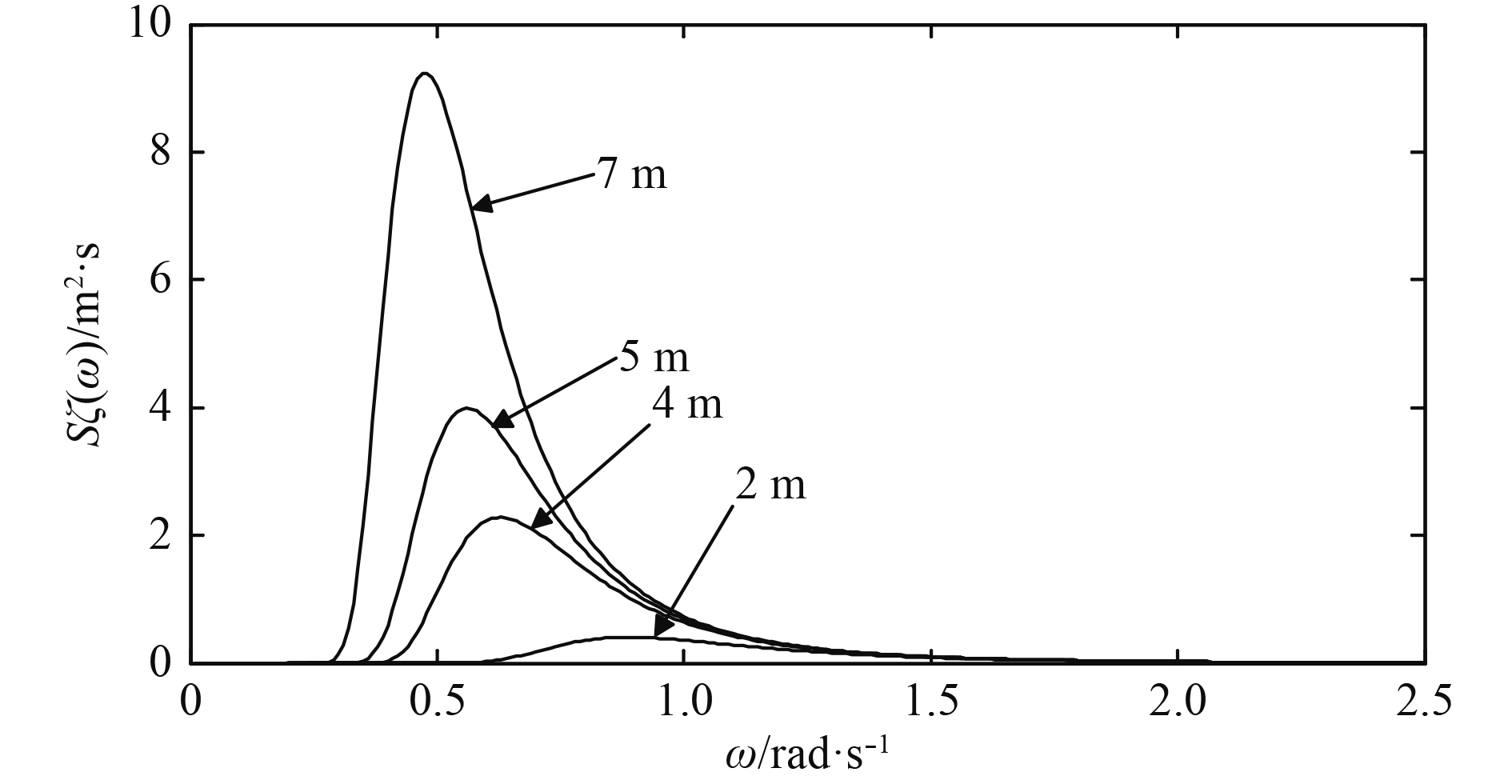图 1 不同有义波高下波能谱密度图 Fig. 1 Waveform density map of different sense wave heights

 ${\alpha _\omega }{\rm{ = }}\frac{{\omega _n^2}}{g}\sum\limits_{n = 1}^{25} {\sqrt {2{S_\zeta }({\omega _n})\Delta \omega } } \cos ({\omega _n}t + {\varepsilon _n}){\text{。}}$ (3)

 ${\omega _e} = \omega \left(1 - \frac{{\omega V}}{g}\cos {\mu _e}\right){\text{。}}$ (4)

 $\begin{split} &{\alpha _{\omega e}} = {K_B}{K_T}{\alpha _\omega }{\text{，}}\\ &{K_B} = 1 - \sqrt {{C_\omega }} {(B/\lambda )^2}{\text{，}}\\ &{K_T} = {e^{ - kd/2}}{\text{。}} \end{split}$ (5)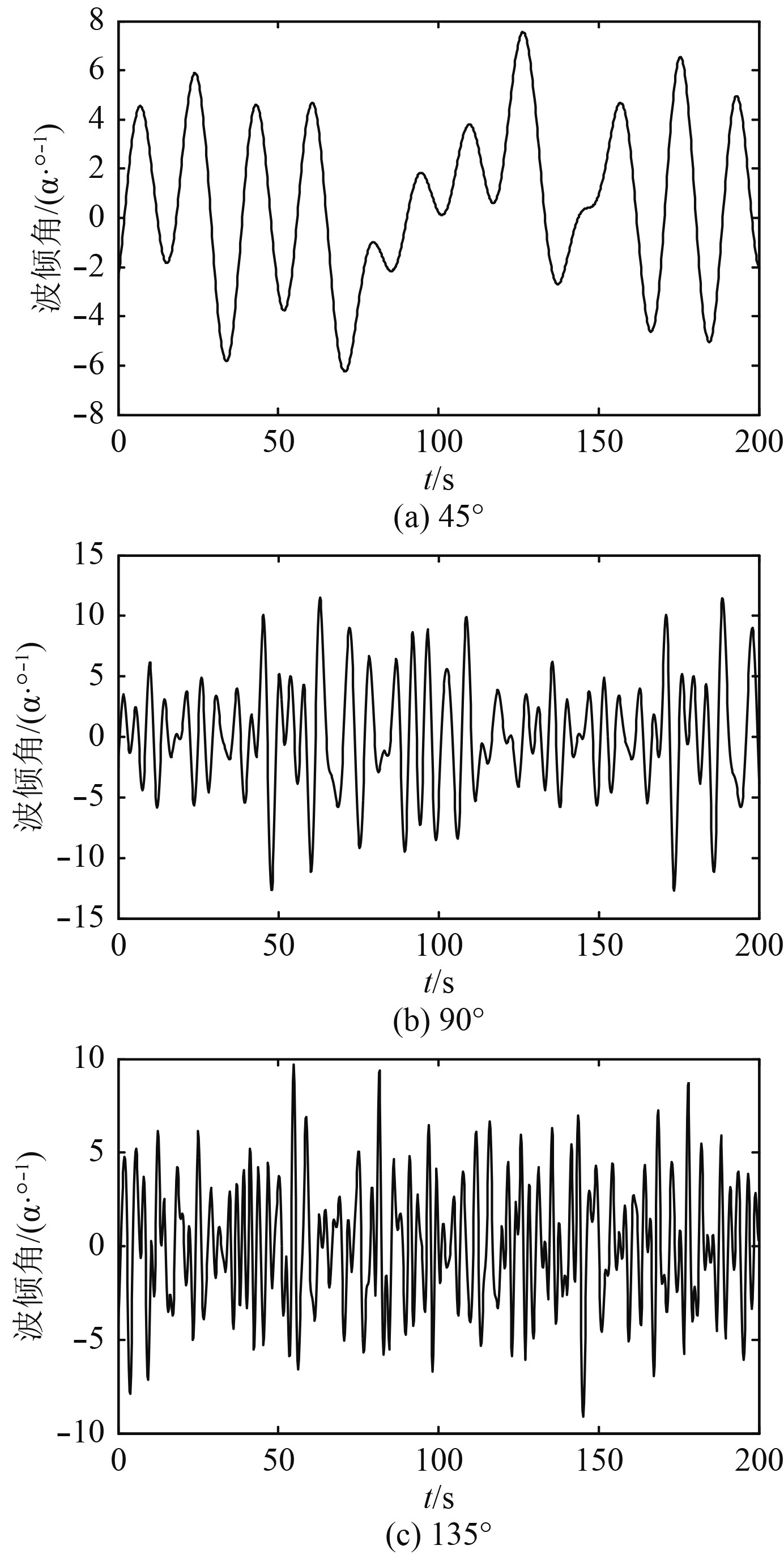图 2 遭遇角分别为45°，90°，135°时的波倾角曲线 Fig. 2 Wave inclination curve of the encounter angles of 45°，90° and 135°
2 船舶及减摇鳍模型

2.1 船舶横摇模型

 $({J_\phi } + \Delta {J_\phi })\ddot \phi + 2{N_\phi }\dot \phi + Dh\phi = - Dh{a_e}{\text{。}}$ (6)

 ${G_\phi }(s) = \frac{{\phi (s)}}{{{a_e}(s)}} = \frac{1}{{T_\phi ^2{s^2} + 2{T_\phi }{\zeta _\phi }s + 1}}{\text{。}}$ (7)

2.2 减摇鳍系统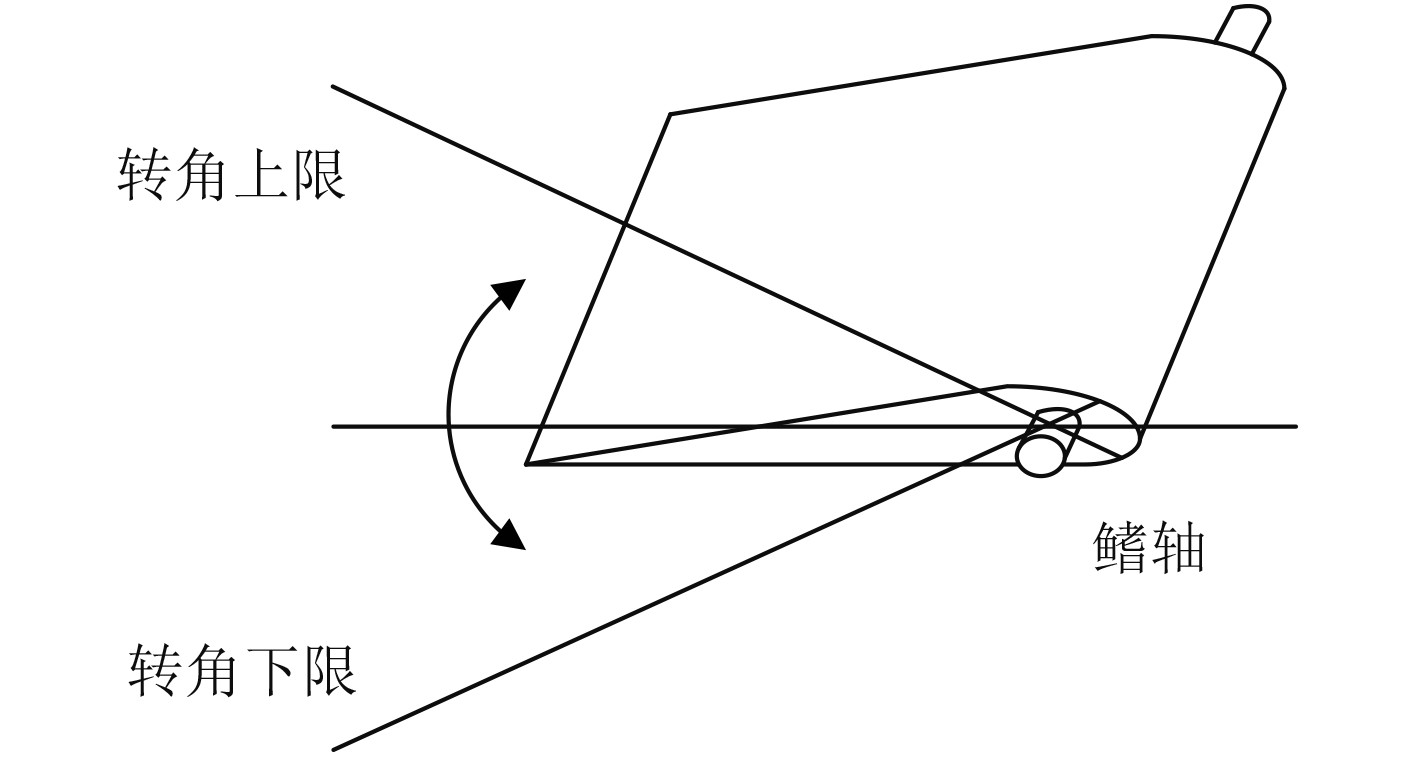图 3 减摇鳍工作方式 Fig. 3 Fins work mode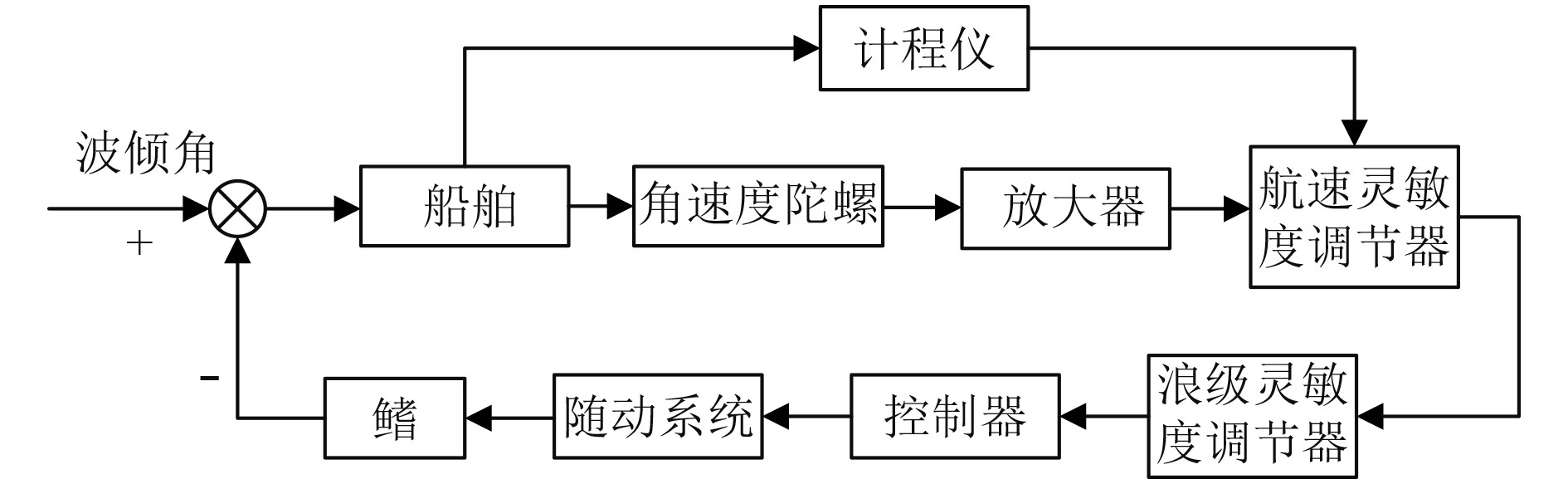图 4 减摇鳍系统原理图 Fig. 4 Schematic diagram of the fin stabilizer system

1）角速度陀螺仪

 ${G_a}(s) = \frac{{400s}}{{{s^2} + 80s + 4\;000}}{\text{。}}$ (8)

2）放大器

 ${G_Q}(s) = {K_Q} = 40{\text{。}}$ (9)

3）航速调节器和浪级调节器

 ${G_H} = {G_L} = 1\text{。}$ (10)

4）控制器

 $\begin{split} & {W_{PID}}(s) = {K_P} + \frac{{{K_I}}}{{{T_I}s + 1}} + \frac{{{K_D}{T_{d1}}s}}{{({T_{d1}}s + 1)({T_{d2}}s + 1)}} =\\ & {K_P} + \frac{{{K_I}}}{{24.607s + 1}} + \frac{{0.064{K_D}s}}{{(0.064s + 1)(0.18s + 1)}} {\text{。}} \end{split}$ (11)

5）随动系统

 ${G_s}(s) = \frac{{550}}{{{s^2} + 15s + 225}}{\text{。}}$ (12)

6）鳍角转换

 ${K_a} = 0.256\;4 {\text{。}}$ (13)
3 控制系统设计 3.1 免疫算法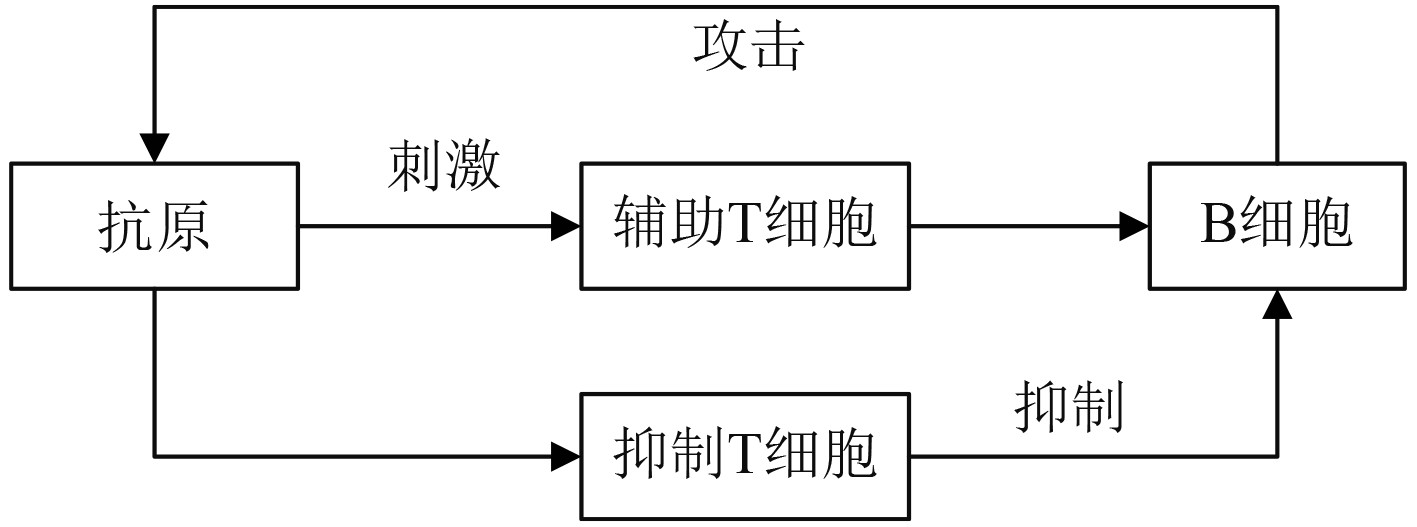图 5 免疫系统原理 Fig. 5 Principle of the immune system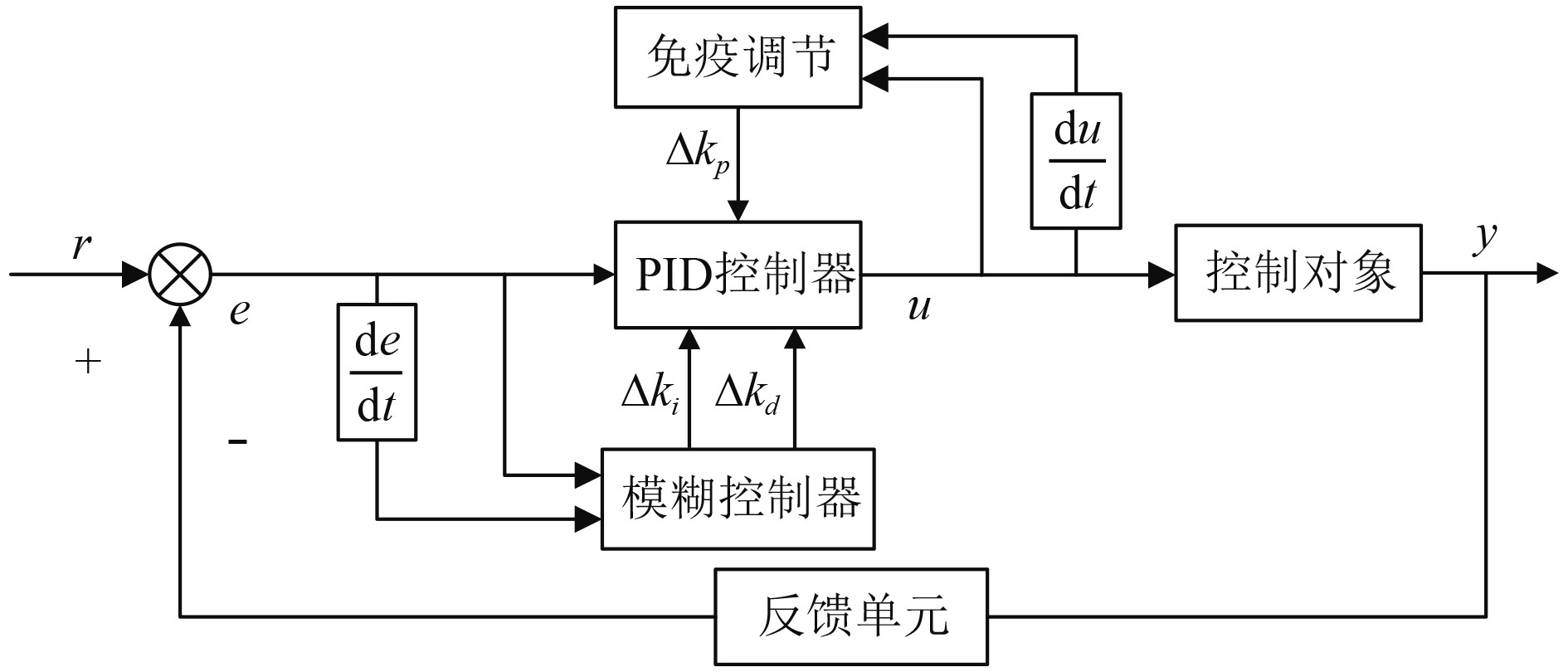图 6 模糊免疫原理图 Fig. 6 Fuzzy immune schematic

 $S(k) = {T_H}(k) - {T_S}(k){\text{。}}$ (14)

 $u(k) = {k_1}[1 - \eta f(u(k),\Delta u(k))]e(k) = {k_p}e(k) {\text{。}}$ (15)

3.2 模糊免疫控制

1）当船体向左偏角及角速度均较大，左鳍需要快速转一较大正攻角，右鳍转相同大小的负攻角；

2）当船体向左偏角较大，角速度向左且偏小，左鳍需要转一较小的正攻角，右鳍转同一角度负攻角；

3）当船体向左偏角较小，角速度向右且偏小，此时使鳍在零位，即不转攻角；

4）当船体向左偏角较小，角速度向右且较大，右鳍转一正攻角，左鳍转一同角度负攻角。表 2 Δkd模糊规则 Tab.2 Table 1 Δkd fuzzy rules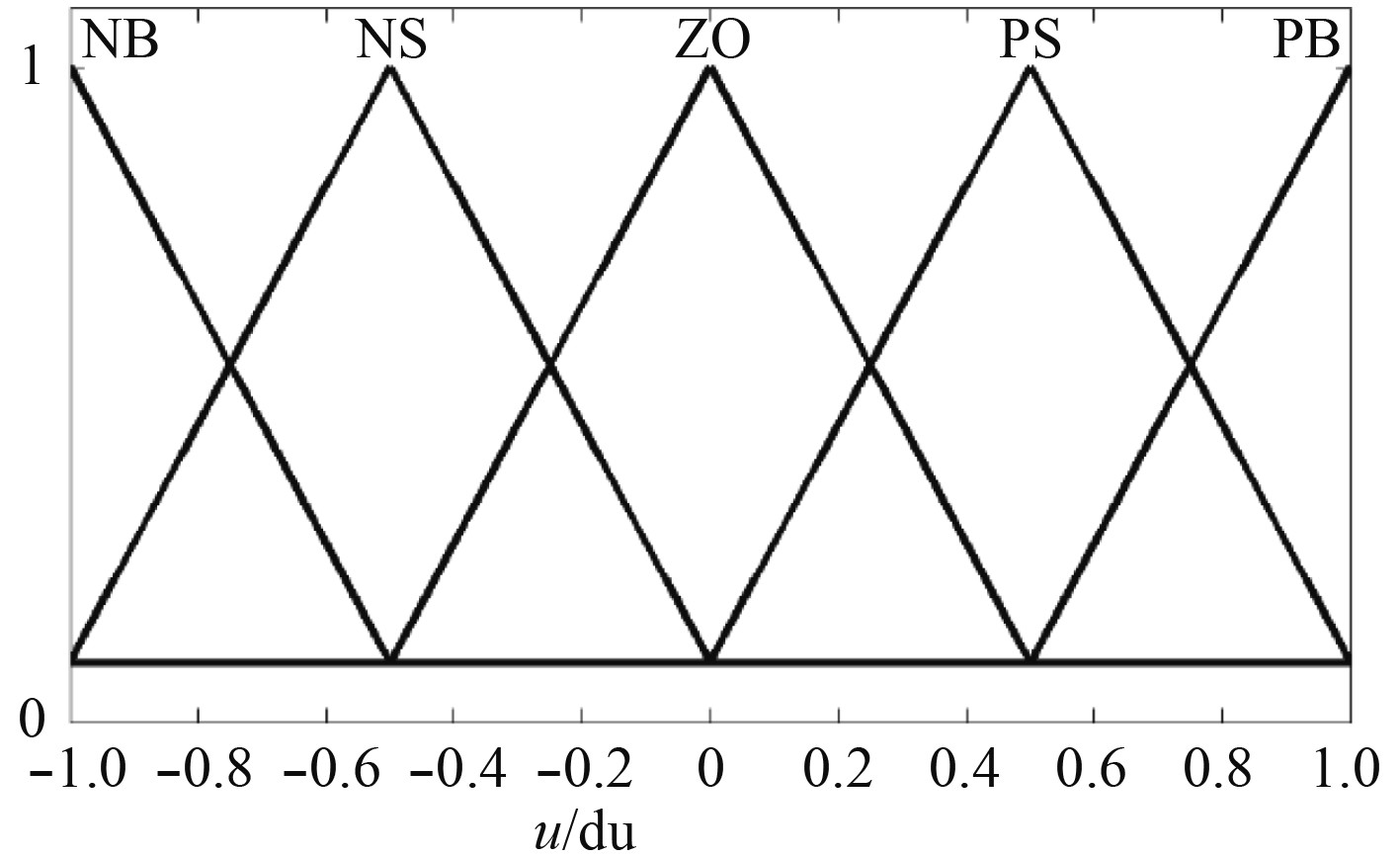图 7 免疫输入隶属度 Fig. 7 Immunological input membership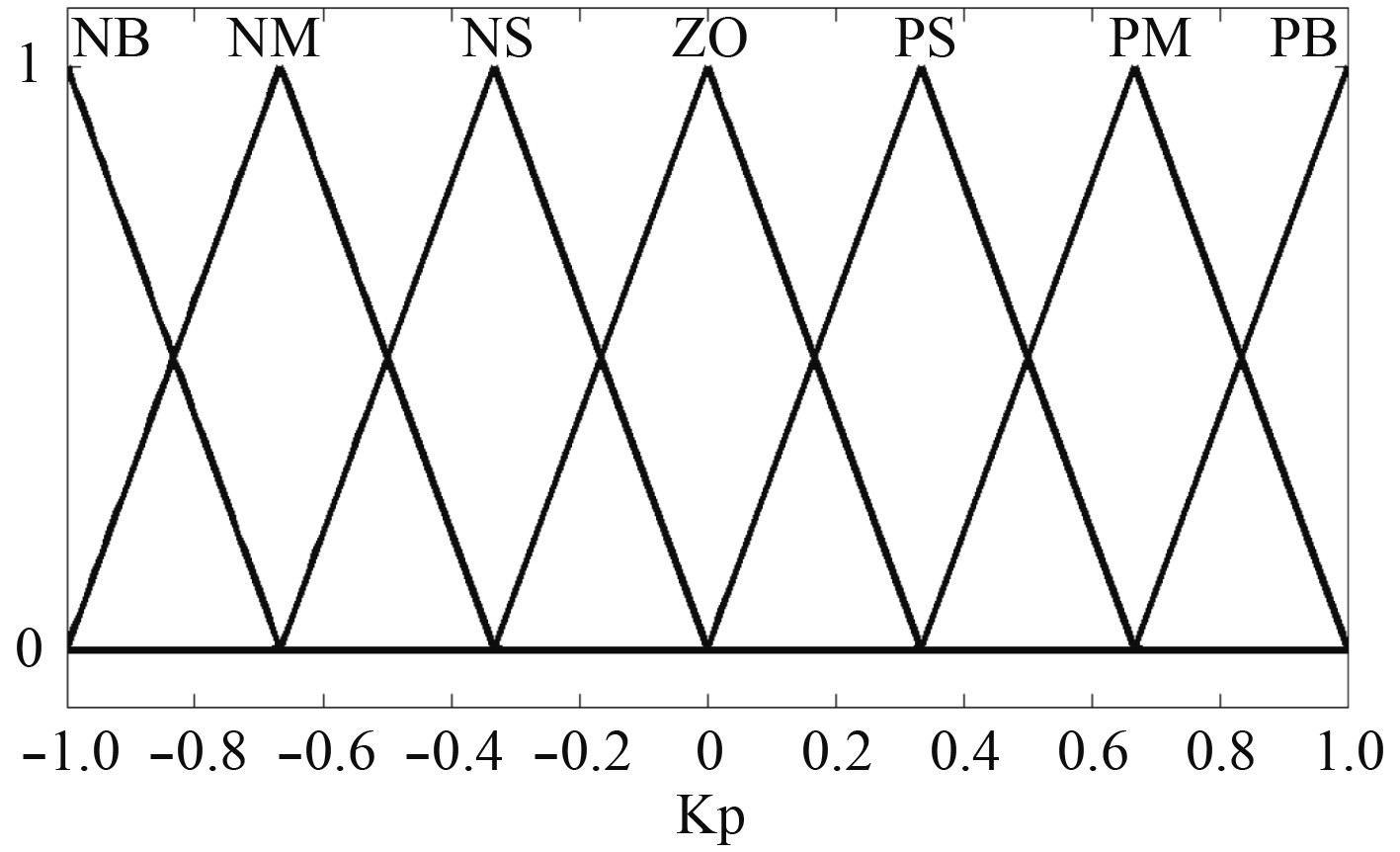图 8 免疫输出隶属度 Fig. 8 Immunity output membership表 1 Δki模糊规则 Tab.1 Δki fuzzy rules表 3 免疫推理规则 Tab.3 Immune inference rules
4 系统仿真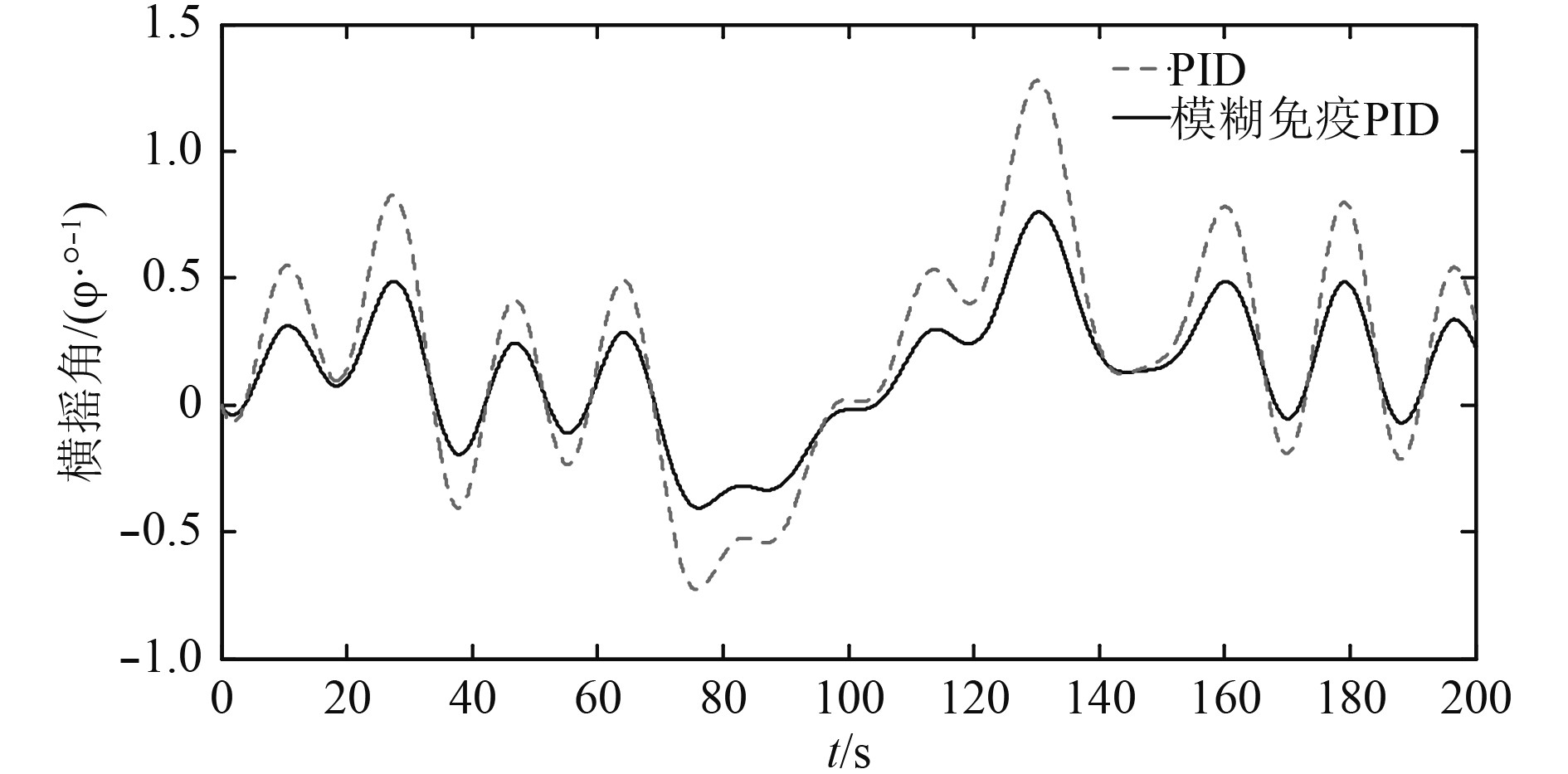图 9 遭遇角为45°横摇角变化曲线 Fig. 9 The rolling angle curve of encounter angle 45°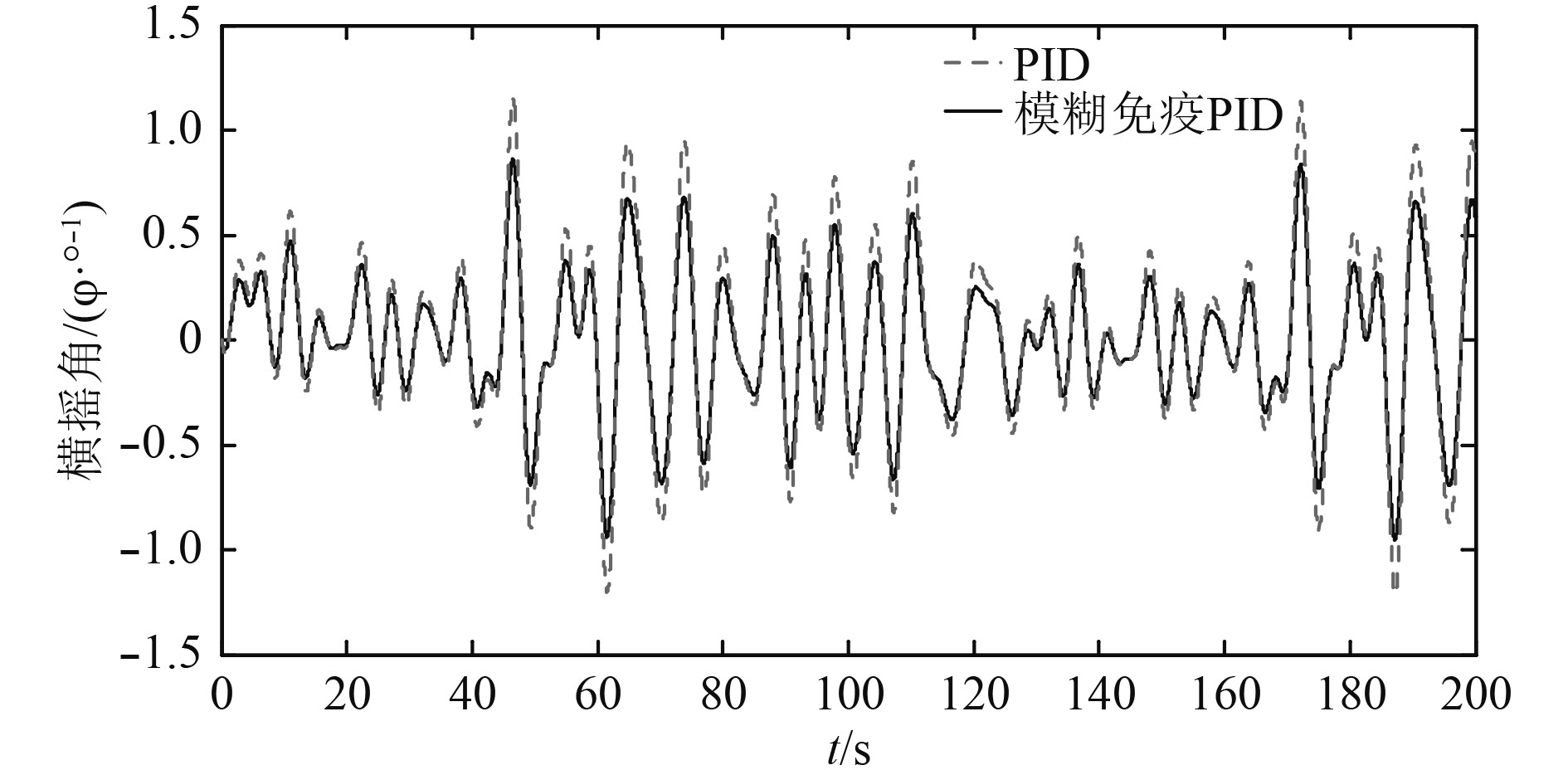图 10 遭遇角为90°横摇角变化曲线 Fig. 10 The rolling angle curve of encounter angle 90°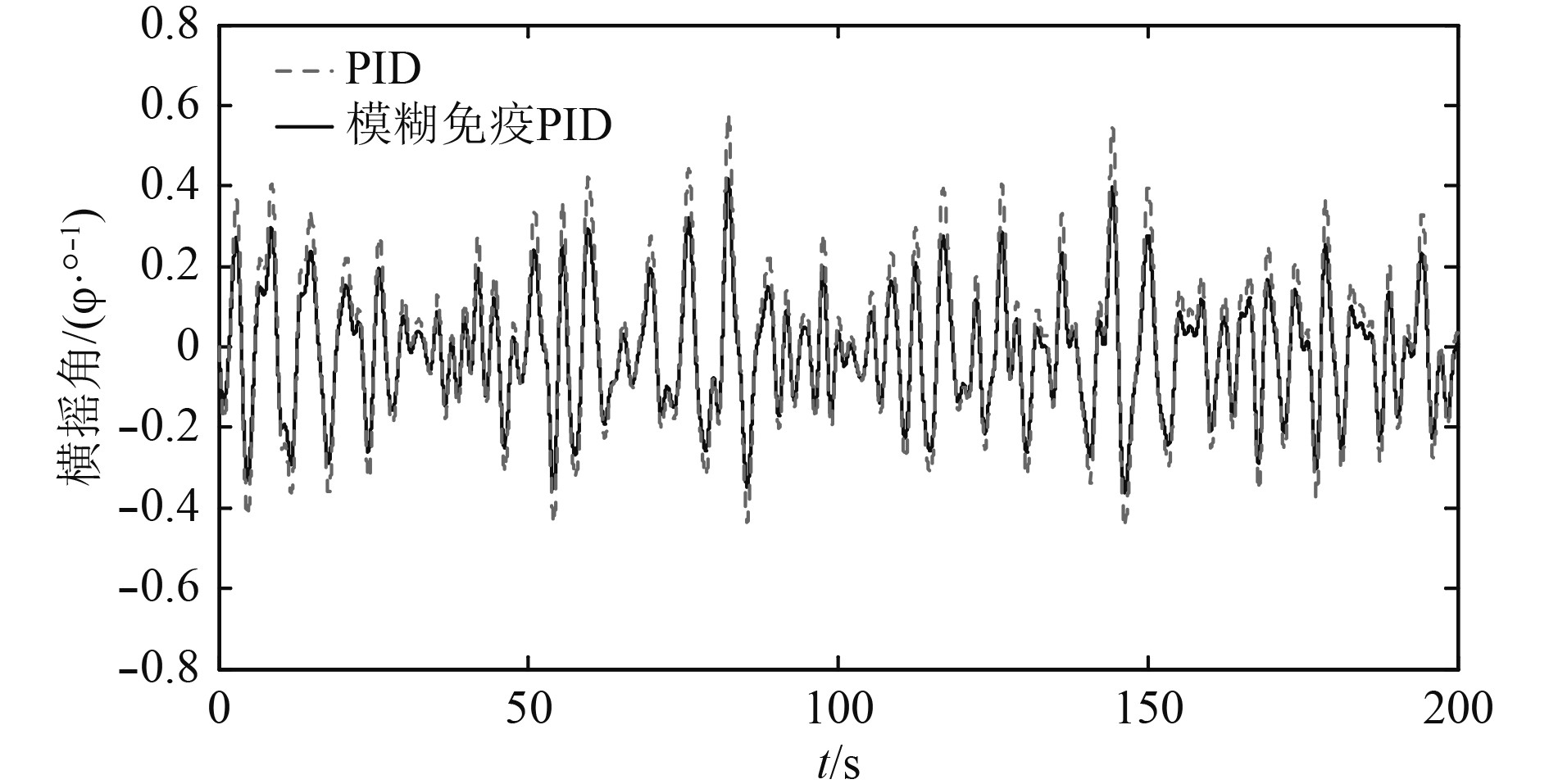图 11 遭遇角为135°横摇角变化曲线 Fig. 11 The rolling angle curve of encounter angle 135°
5 结　语

  郑则炯, 梁文祯. 基于变结构鲁棒性控制的船舶减摇鳍非线性系统研究[J]. 舰船科学技术, 2017, 39(22): 46-48.  刘志全, 金鸿章. 基于航速保持的舵减摇控制方法[J]. 中国舰船研究, 2017, 12(1): 128-133. DOI:10.3969/j.issn.1673-3185.2017.01.019  张树豪. 基于自抗扰控制的船舶横摇控制研究[D]. 大连: 大连海事大学, 2016.  田军. 舵鳍联合控制参数优化及仿真[J]. 自动化技术与应用, 2015, 34(7): 9-13.  Q. Zhigang, L. Yanwen J. Hong, Research on the lift characteristic of morphing bionic fin stabilizer at zero/low speed[C]// 2015 IEEE International Conference on Mechatronics and Automation (ICMA), Beijing, 2015, 1374-1378.  闫爱平, 王帆. 减摇鳍系统自适应反演滑膜控制的设计及仿真研究[J]. 舰船科学技术, 2016, 38(12): 16-18.  王辉, 王科俊, 于立君. 减摇鳍模糊免疫自适应PID控制器设计及仿真研究[J]. 海军工程大学学报, 2007(4): 17-21+29. DOI:10.3969/j.issn.1009-3486.2007.04.005  段玉波, 马杰. 模糊免疫PID控制器设计及其仿真研究[J]. 自动化技术与应用, 2017, 36(4): 106-110.  梁利华, 赵朋, 张松涛, 等. 基于相位匹配的零航速减摇鳍控制策略研究[J]. 哈尔滨工程大学学报, 2018, 39(9): 1539-1545.